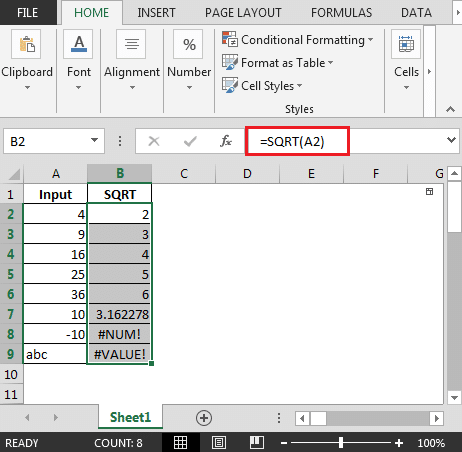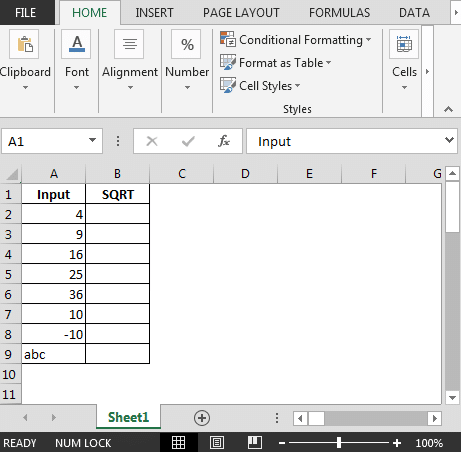# If you want to calculate the square root of a number in Excel, you can use the square formula. In this article, we will learn how to take the square root of a number.

You can use the SQRT function in versions: Excel2013 , Excel2010 , Excel2007 , Excel2003 .

To do this, follow these steps:• In cell B2, write the formula (SQRT (A2 = ).
• By putting the formula in other cells, we get the square root of the numbers in Excel.A few things to consider:

• Cell B8 Error #NUM! is showing.
• The reason for this error is the existence of a negative number.
• Cell B9 # VALUE ! is showing.
• The reason for this error is that the value entered can not be counted as a number.

With this method, you can calculate the square of numbers in Excel.